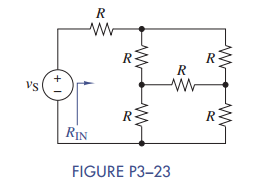### Create an Account

Already have account?

### Forgot Your Password ?

Home / Questions / Use simple engineering intuition to find the input resistance of the circuit in Figure ...

# Use simple engineering intuition to find the input resistance of the circuit in Figure P3 23 Use either node-voltage or mesh-current analysis to prove your

Use simple engineering intuition to find the input resistance of the circuit in Figure P3−23. Use either node-voltage or mesh-current analysis to prove your intuition.Jun 15 2020 View more View Less

#### Answer (Solved)Subscribe To Get Solution